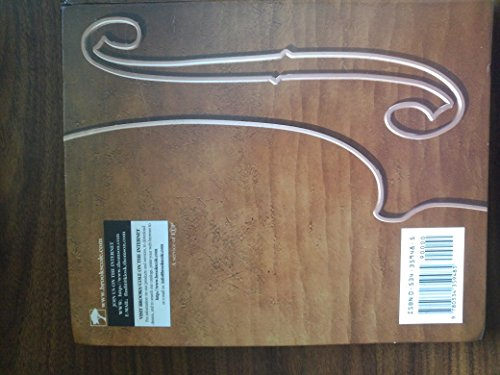# Multivariable Calculus by James Stewart

In Stock
\$20.99
+10% GST
A reprinting of the multivariable portion of "Calculus, Fourth Edition". These reproduced chapters cover: vectors and the geometry of space, vector functions, partial derivatives, multiple integrals, vector calculus, and second-order differential equations.
Only 1 left

## Multivariable Calculus Summary

### Multivariable Calculus by James Stewart

A reprinting of the multivariable portion of "Calculus, Fourth Edition". These reproduced chapters cover: vectors and the geometry of space, vector functions, partial derivatives, multiple integrals, vector calculus, and second-order differential equations. These chapters are a direct continuation of "Single Variable Calculus, Fourth Edition" and "Single Variable Calculus, Early Transcendentals, Fourth Edition". Particularly important results from single variable calculus are summarized for at-a-glance review.

### Why buy from World of BooksOur excellent value books literally don't cost the earthFree delivery in AustraliaEvery used book bought is one saved from landfill

11. PARAMETRIC EQUATIONS AND POLAR COORDINATES. Curves Defined by Parametric Equations. Tangents and Areas. Arc Length and Surface Area. Polar Coordinates. Areas and Lengths in Polar Coordinates. Conic Sections. Conic Sections in Polar Coordinates. Review. Problems Plus. 12. INFINITE SEQUENCES AND SERIES. Sequences. Series. The Integral Test and Estimates of Sums. The Comparison Tests. Alternating Series. Absolute Convergence and the Ratio and Root Tests. Strategy for Testing Series. Power Series. Representation of Functions as Power Series. Taylor and Maclaurin Series. The Binomial Series. Applications of Taylor Polynomials. Review. Problems Plus. 13. VECTORS AND THE GEOMETRY OF SPACE. Three-Dimensional Coordinate Systems. Vectors. The Dot Product. The Cross Product. Equations of Lines and Planes. Cylinders and Quadric Surfaces. Cylindrical and Spherical Coordinates. Review. Problems Plus. 14. VECTOR FUNCTIONS. Vector Functions and Space Curves. Derivatives and Integrals of Vector Functions. Arc Length and Curvature. Motion in Space: Velocity and Acceleration. Review. Problems Plus. 15. PARTIAL DERIVATIVES. Functions of Several Variables. Limits and Continuity. Partial Derivatives. Tangent Planes and Differentials. The Chain Rule. Directional Derivatives and the Gradient Vector. Maximum and Minimum Values. Lagrange Multipliers. Review. Problems Plus. 16. MULTIPLE INTEGRALS. Double Integrals over Rectangles. Iterated Integrals. Double Integrals over General Regions. Double Integrals in Polar Coordinates. Applications of Double Integrals. Surface Area. Triple Integrals. Triple Integrals in Cylindrical and Spherical Coordinates. Change of Variables in Multiple Integrals. Review. Problems Plus. 17. VECTOR CALCULUS. Vector Fields. Line Integrals. The Fundamental Theorem for Line Integrals. Green's Theorem. Curl and Divergence. Parametric Surfaces and Their Areas. Surface Integrals. Stokes' Theorem. The Divergence Theorem. Summary. Review. Problems Plus. 18. SECOND-ORDER DIFFERENTIAL EQUATIONS. Second-Order Linear Equations. Nonhomogeneous Linear Equations. Applications of Second-Order Differential Equations. Series Solutions. Review. Problems Plus. APPENDIXES. Appendix F: Proofs of Theorems. Appendix G: Complex Numbers. Appendix H: Answers to Odd-Numbered Exercises.

GOR002627188
Multivariable Calculus by James Stewart
James Stewart
Used - Very Good
Hardback
Cengage Learning, Inc
1999-05-31
450
0534359485
9780534359485
N/A
Book picture is for illustrative purposes only, actual binding, cover or edition may vary.
This is a used book - there is no escaping the fact it has been read by someone else and it will show signs of wear and previous use. Overall we expect it to be in very good condition, but if you are not entirely satisfied please get in touch with us.